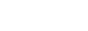## How do I extract hours, minutes, or seconds from a time?

Short answer: Times are integers (think milliseconds).

```q)(`hh`mm`ss \$ .z.T), `int \$ .z.T mod 1000
16 49 2 233
q)
```

You can extract the hours, minutes, and seconds from a time by passing `hh, `mm, and `ss, respectively, as left arguments to \$ (cast):

```q)now: .z.T
q)now
16:49:02.233
q)`hh \$ now
16
q)`mm \$ now
49
q)`ss \$ now
2
q)`hh`mm`ss \$ now
16 49 2
q)
```

Getting the milliseconds from a time is slightly less obvious. Times (type -19) are represented internally by q as 32-bit integers; typically the value counts the number of milliseconds since midnight, but it can also represent a span of time. We can cast freely back and forth between the two types and the values are preserved:

```q)`time \$ 0
00:00:00.000
q)`int \$ 00:00:00.000
0q)`time \$ 24 * 60 * 60 * 1000
24:00:00.000
q)`int \$ 24:00:00.000
86400000
q)`int \$ now
60542233
q)`time \$ 60542233
16:49:02.233
q)
```

Not only is the internal representation of time simply an integer, we can mix integers and times in integer arithmetic operations, and the result is always a time:

```q)01:00:00.000 + 00:01:00.000
01:01:00.000
q)01:00:00.000 + 60000
01:01:00.000
q)01:00:00.000 * 4
04:00:00.000
q)now - 01:30:20.123
15:18:42.110
q)
```

By using div and mod, then, we have an alternative means to calculate the components of a time:

```q)now div 3600000 // milliseconds per hour
00:00:00.016
q)now mod 1000    // just the milliseconds, please
00:00:00.233
q)
```

Although extracting milliseconds from a time while keeping the time type (as in the second example above) is sometimes useful, we normally want to get back these components of a time as integers, so let’s cast it:

```q)`int \$ now mod 1000
233
q)
```

By the way, there is a shortcut for getting hours, minutes, and seconds from global variables that hold times: dot notation.

```q)x: .z.T
q)x.hh, x.mm, x.ss
16 49 2
q)
```

However, we rarely use global variables to hold time values.

## How can I measure the performance of my q code?

The simplest way to time something in q is to use the \t command:

```q)f: {[] do[100000; 2 + 2]}
q)\t f[]
10
q)
```

\t executes its argument and returns the number of milliseconds that elapsed – i.e., wall clock time (except when you are using \t in its other sense, i.e., setting or getting the time between timer events).

When using kdb as a database server, you will want to use one or more of Simon’s logging scripts as a starting point. These tremendously useful scripts enable you to record every incoming query as well as measure the time taken by every incoming query. That way you can calculate summary statistics on actual database performance in order to find and fix intermittent problems.## How do I extract the milliseconds from a time?

mod 1000:

q)now: .z.T
q)now
00:15:00.812
q)now mod 1000
00:00:00.812

If you want the milliseconds as an integer, you can simply follow the mod with a conversion to int:

q)`int\$ now mod 1000
812

You don’t want to pass a datetime to mod; the result you’ll get is not what you had in mind. You must convert it to a time first:

q)now: .z.Z
q)now
2011.03.26T09:51:26.624
q)now mod 1000
102.4107
q)`int\$ (`time\$ now) mod 1000
624
q)